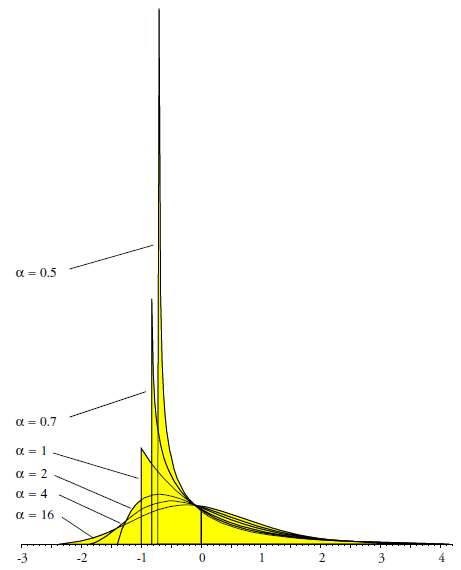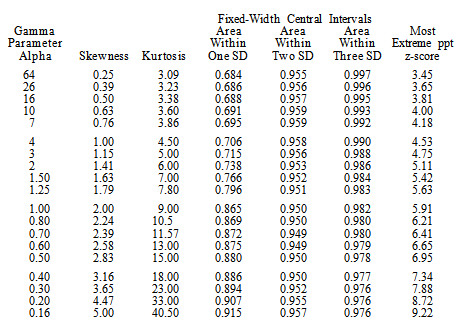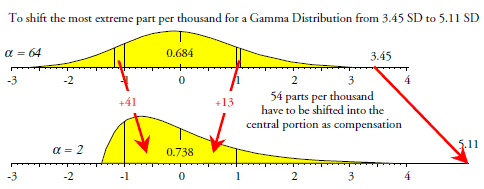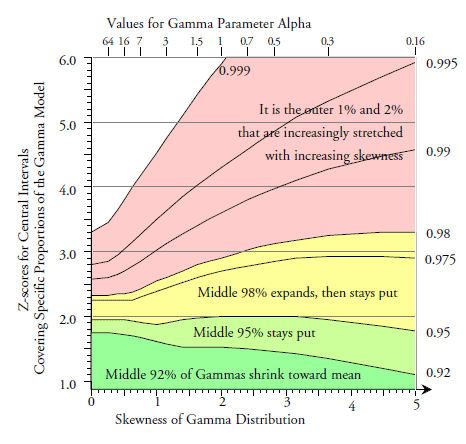#Quality Digest

Featured Product
This Week in Quality Digest Live
Six Sigma Features
Donald J. Wheeler
Using process behavior charts in a clinical setting
Alan Metzel
Introducing the Enhanced Perkin Tracker
Donald J. Wheeler
What you think you know may not be so
William A. Levinson
The AIAG offers a clearly defined and powerful synergy between the three
Donald J. Wheeler
How does it compare with a process behavior chart?
Six Sigma News
Sept. 28–29, 2022, at the MassMutual Center in Springfield, MA
Elsmar Cove is a leading forum for quality and standards compliance
Is the future of quality management actually business management?
Too often process enhancements occur in silos where there is little positive impact on the big picture
Collect measurements, visual defect information, simple Go/No-Go situations from any online device
Good quality is adding an average of 11 percent to organizations’ revenue growth
Floor symbols and decals create a SMART floor environment, adding visual organization to any environment
A guide for practitioners and managers
Making lean Six Sigma easier and adaptable to current workplacesBio

Six Sigma

## Properties of Probability Models, Part 2

### What they forgot to tell you about the Gammas

Published: Tuesday, September 1, 2015 - 15:53

Story update 9/8/2015: There was an error in the data set for columns "Skew" and "Kurt" in figure 7. The error has been corrected.

Clear thinking and simplicity of analysis require concise, clear, and correct notions about probability models and how to use them. Last month in part one we looked at the properties of the Weibull probability models and discovered that some ideas about skewed distributions are incorrect. Here we shall examine the basic properties of the family of Gamma models.

How would you characterize a skewed distribution? When asked this question most will answer, "A skewed distribution is one that has a heavy, elongated tail." This idea is expressed by saying that a distribution becomes more heavy-tailed as its skewness and kurtosis increase. Last month, for Weibull models at least, we discovered that as the tail was elongated it grew lighter, not heavier. Does this happen with other families of probability models? Here we consider the Gamma models.

### The gamma family of distributions

Gamma distributions are widely used in all areas of statistics and are found in most statistical software. Since software facilitates our use of the Gamma models, the following formulas are given in the interest of clarity. Gamma models depend upon two parameters, once again denoted by alpha, α, and beta, β. The probability density function for the Gamma family has the form:The alpha parameter determines the shape of the Gamma model. When the value for alpha is 1.00 or less, the Gamma distributions will be J-shaped. As the value for alpha increases above 1.00, the Gamma distributions become mound-shaped, and as the value for alpha gets large, the Gammas approach the normal distribution. Since we consider these distributions in standardized form, the value for the beta parameter will not affect any of the following results. Six standardized Gamma distributions are shown in figure 1.Figure 1: Six standardized gamma distributions

So what is changing as you select different Gamma probability models? To answer this question, figure 2 considers 19 different Gamma models. For each model we have the skewness and kurtosis, the areas within fixed-width central intervals (encompassing one, two, and three standard deviations on either side of the mean), and the z-score for the 99.9th percentile of the model.

The z-scores in the last column of figure 2 would seem to validate the idea that increasing skewness corresponds to elongated tails. As the skewness gets larger, the z-score for the most extreme part per thousand also increases. This may be seen in figure 3, which plots the skewness vs. the z-scores for the most extreme part per thousand. So skewness is directly related to elongation, as is commonly thought. But what about the weight of the tails?Figure 2: Characteristics for various gamma modelsFigure 3: Skewness and elongation for gamma models

Figure 4 plots the areas for the fixed-width central intervals against the skewness of models from figure 2. The bottom curve of figure 4 (k = 1) shows that the areas found within one standard deviation of the mean of a Gamma distribution increase with increasing skewness. Since the tails of a probability model are traditionally defined as those regions that are more than one standard deviation away from the mean, the bottom curve of figure 4 shows us that the areas in the tails must decrease with increasing skewness. This contradicts the common notion about skewness and a heavy tail.Figure 4: How the coverages vary with skewness for gamma distributions

So while the infinitesimal areas under the extreme tails will move further away from the mean with increasing skewness, the classically defined tails do not get heavier. Rather, they actually get much lighter with increasing skewness. To move the outer few parts per thousand further away from the mean, you have to compensate by moving a much larger percentage closer to the mean. This compensation is unavoidable and inevitable. To stretch the long tail, you have to pack an ever-increasing proportion into the center of the distribution!Figure 5: How the tails get lighter with skewness for Gamma distributions

So while skewness is associated with one tail being elongated, that elongation does not result in a heavier tail, but rather in a lighter tail. Increasing skewness is rather like squeezing toothpaste up to the top of the tube: While concentrating the bulk at one end, little bits get left behind and are squeezed down toward the other end. As these little bits become more isolated from the bulk, the "tail" becomes elongated.

However, once again, there are a couple of surprises about this whole process. The first of these is the middle curve of figure 4 (k = 2), which shows the areas within the fixed-width, two-standard-deviation central intervals. The flatness of this curve shows that the areas within two standard deviations of the mean of a Gamma stay around 95 percent to 96 percent regardless of the skewness.

In statistics classes students are taught that having approximately 95 percent within two standard deviations of the mean is a property of the normal distribution. Last month we found that this was a property of the family of Weibull models. Here we see that this property also applies to the Gamma distributions. Beginning with the mound-shaped Gammas and continuing through the J-shaped Gammas, there will be approximately 95 percent to 96 percent within two standard deviations of the mean.

The second unexpected characteristic of the Gammas is seen in the top curve of figure 4 (k = 3), which shows the areas within the fixed-width, three-standard-deviation central intervals. While the area within three standard deviations of the mean does drop slightly at first, it stabilizes for the J-shaped Gammas at about 97.5 percent. This means that a fixed-width, three-standard-deviation central interval for a Gamma distribution will always contain at least 97.5 percent of that distribution.Figure 6: What gamma distributions have in common

So if you think your data are modeled by a Gamma distribution, then even without any specific knowledge as to which of the Gamma distributions is appropriate, you can safely say that 97.5 percent or more will fall within three standard deviations of the mean, and that approximately 95 percent or more will fall within two standard deviations of the mean. Fitting a particular Gamma probability model to your data will not change either of these statements to any practical extent.

For many purposes these two results will be all you need to know about your Gamma model. Without ever actually fitting a Gamma probability model to your data, you can filter out either 95 percent or 98 percent of the probable noise using generic, fixed-width central intervals.

### What gets stretched?

If the tail gets both elongated and thinner at the same time, something has to get stretched. To visualize how skewness works for Gamma models we can compare the widths of various fixed-coverage central intervals. These fixed-coverage central intervals will be symmetrical intervals of the form:

MEAN(X) ± Z SD(X)

While this looks like the formula for the earlier fixed-width intervals, the difference is in what we are holding constant and what we are comparing. When we hold the widths fixed, we compare the areas covered by the intervals. When we hold the coverages fixed, we compare the widths of the intervals. These widths are characterized by the z-scores in figure 7. For example, a Gamma model with an alpha parameter of 1.25 will have 92 percent of its area within 1.53 standard deviations of the mean, and it will have 99 percent of its area within 3.49 standard deviations of the mean.Figure 7: Widths of fixed-coverage central intervals for gamma models

Figure 8 shows the values in each column of figure 7 plotted against skewness. The bottom curve shows that the middle 92 percent of a Gamma will shrink with increasing skewness. The 95-percent fixed-coverage intervals are remarkably stable until the increasing mass near the mean eventually begins to pull this curve down. The 97.5-percent fixed-coverage intervals initially grow until they plateau near three standard deviations.Figure 8: Widths of fixed-coverage central intervals for Gamma models

The spread of the top three curves shows that for the Gamma models, it is primarily the outermost 2 percent that gets stretched into the extreme upper tail. While 920 parts per thousand are moving toward the mean, and while another 60 parts per thousand get slightly shifted outward and then stabilize, it is primarily the outer 20 parts per thousand that bear the brunt of the stretching and elongation that goes with increasing skewness.

### The benefits of fitting a gamma distribution

So what do you gain by fitting a Gamma model to your data? The value for the alpha parameter may be estimated from the average and standard deviation statistics, and this estimate will, in turn, determine the shape of the specific Gamma model you fit to your data. Since these statistics will be more dependent upon the middle 95 percent of the data than the outer one percent or less, you will end up primarily using the middle portion of the data to choose a Gamma model. Since the tails of a Gamma model become lighter with increasing skewness, you will end up making a much stronger statement about how much of the area is within one standard deviation of the mean than about the size of the elongated tail. Fitting a Gamma distribution is not so much about the tails as it is about how much of the model is found within one standard deviation of the mean. So, while we generally think of fitting a model as matching the elongated tail of a histogram, the reality is quite different.

Once you have a specific Gamma model, you can then use the model to extrapolate out into the extreme tail (where you are unlikely to have any data) to compute critical values that correspond to infinitesimal areas under the curve. However, as may be seen in figures 3 and 8, even small errors in estimating the parameter alpha can have a large impact upon the critical values computed for the infinitesimal areas under the extreme tail of your Gamma model. As a result, the critical values you compute for the upper one or two percent of your Gamma model will have virtually no contact with reality. Such computations will always be more of an artifact of the model used than a characteristic of either the data or the process that produced the data.

To illustrate this point, I generated 5,000 data sets of 100 values each using an exponential distribution (which is a Gamma with an alpha parameter of 1.000). For each data set I estimated the value of alpha. These estimates ranged from 0.495 to 2.103. From figure 3 we can see that this range of values for alpha will result in Gamma models that have their most extreme part per thousand anywhere in the range from five SD to seven SD above the mean. Thus, the uncertainty in your estimate of the alpha parameter will create large uncertainties in the location of the infinitesimal areas under the extreme tail. Consequently, any extreme tail critical values you compute will be more of an artifact of your model than a characteristic of your data.

### Industrial data analysis

What impact does all this have on how we analyze data? It turns out that there are two distinctly different approaches to data analysis. For clarity call these the statistical approach and Shewhart's approach.

The statistical approach uses fixed-coverage intervals for the analysis of experimental data. In some cases these fixed-coverage intervals are not centered on the mean, but rather involve fixed coverages for the tail areas, but this is still analogous to the fixed-coverage central intervals used above. Fixed coverages are used because experiments are designed and conducted to detect specific signals, and we want the analysis to detect these signals despite the noise present in the data. By using fixed coverages, statisticians can fine-tune just how much of the noise is being filtered out. This fine-tuning is important because additional data are not generally going to be available, and we need to get the most out of the limited amount of experimental data. Thus, the complexity and cost of most experiments will justify a fair amount of complexity in the analysis. Moreover, to avoid missing real signals within the experimental data, it is traditional to filter out only 95 percent of the probable noise.

Shewhart's approach was created for the continuing analysis of observational data that are the byproduct of operations. To this end Shewhart used a fixed-width interval rather than a fixed-coverage interval. His argument was that we will never have enough data to ever fully specify a particular probability model for the original data. Moreover, since additional data will typically be available, we do not need to fine-tune our analysis—the exact value of the coverage is no longer critical. As long as the analysis is reasonably conservative, it will allow us to find those signals that are large enough to be of economic importance without getting too many false alarms. So, for the real-time analysis of observational data, Shewhart chose to use a fixed-width, three-sigma central interval. As we have seen, such an interval will routinely filter upwards of 98 percent of the probable noise.Figure 9: How three-sigma limits work with Gamma distributions

What we have discovered here is that Shewhart's simple, generic, three-sigma limits will provide a conservative analysis for any and every data set that might logically be considered to be modeled by a Gamma distribution. Last month we discovered that Shewhart's simple, generic, three-sigma limits also provide a conservative analysis for any and every data set that might logically be considered to be modeled by a Weibull distribution. This is why finding exact critical values for a specific probability model is not a prerequisite for using a process behavior chart. Once you filter out approximately 98 percent or more of the probable noise, anything left over is a potential signal.

### Discuss### Donald J. Wheeler

Find out about Dr. Wheeler’s virtual seminars for 2023 at www.spcpress.com. Dr. Wheeler is a fellow of both the American Statistical Association and the American Society for Quality who has taught more than 1,000 seminars in 17 countries on six continents. He welcomes your questions; you can contact him at djwheeler@spcpress.com.

### Which sigma are we using to bound 98% ?

Are we wrapping up 98% or more of the data within 3 standard deviations computed the typical way (sample standard deviation calculation with squared differences from the mean, summed, n minus one divisor, square root) or through a moving range estimate? I mention moving range since the article invokes Shewhart. I've seen plenty of datasets in which 98% or more of the data are within 3 standard deviations of the mean using the typical calculation but more than 2% of the results are beyond 3-sigma (MR-based) limits.

Thanks.

### Rational subgroup issue

If the two estimates are not roughly equal, the rational subgroup has not been defined correctly. This is when Cpk >> Ppk.

### I'm interested in individual-x/mr results

I'm trying to understand whether Dr. Wheeler expects this 98% notion to hold up when running individuals charts. He's obviously a big fan of such charts, so it seems like a reasonable question.

My point is that a moving-range estimate of the standard deviation is likely to be less than the (global) estimate of standard deviation for skewed data. Try it in your favorite software package -- generate some lognormal data and compare the (global) standard deviation with the moving-range estimate. Isn't d2 (1.128 for MR of 2) based on a normal population?

Note from Dr. Wheeler's column dated February 2010: There are only two measures of dispersion to use when creating a chart for individual values: The average moving range and the median moving range ... The use of any other measure of dispersion is wrong.

Thanks.

### Maximum likelihood is better

Re: "The value for the alpha parameter may be estimated from the average and standard deviation statistics, and this estimate will, in turn, determine the shape of the specific Gamma model you fit to your data. Since these statistics will be more dependent upon the middle 95 percent of the data than the outer one percent or less, you will end up primarily using the middle portion of the data to choose a Gamma model."

This is true because the average and standard deviation are functions of alpha and gamma, so we have 2 equations with 2 variables. The problem with this approach is, however, exactly the one Dr. Wheeler points out. The maximum likelihood method is much better, although it requires iterative methods to determine alpha and gamma. The estimates from the average and standard deviation are good starting guesses, but they rarely equal the (best) final results.

Another problem with using the average and standard deviation to find the parameters is that you cannot then find the threshold parameter for a 3-parameter gamma model. This has to be done with a double-iterative process that optimizes the threshold parameter (delta), which in turn requires maximum likelihood estimation of alpha and gamma for each delta.

### It is not about the method of estimation

The point is not about how we find an estimate of the parameters for a probability model.  But rather that regardless of how we estimate our parameters, the whole process is filled with uncertainty, and that these uncertainties will have the greatest impact upon the extreme critical values.  The statistical approach and Shewhart's approach are diametrically opposite, and until someone understands this, they cannot begin to understand how Shewhart's distribution free approach can work.

### More than logic needed

A great paper as always but most will never grasp it.  More importantly, those in power making decisions will never even bother to understand it.  It is safer for their careers to simply follow the herd.

I've always seen parallels with the \$1.5T pa global warming scam.  There is zero evidence of any kind to support the idea that man's CO2 is causing a global warming that stopped 2 decades ago, yet incredibly, people still believe.  Science and logic is not enough to sway the masses.  Unlike quality, the next ice age will show just how gullible the believers have been.  In quality, no amount of logic alone will bring the majority back to Shewhart.

### The cap and trade scam

http://www.telegraph.co.uk/news/earth/copenhagen-climate-change-confe/67... shows just how much the people who are pushing carbon emission regulations really believe in man-made global warming.

"The airport says it is expecting up to 140 extra private jets during the peak period alone, so far over its capacity that the planes will have to fly off to regional airports – or to Sweden – to park, returning to Copenhagen to pick up their VIP passengers."

Sort of like the CEO who talks about the importance of quality, and then responds to a nonconformance report by telling the people to ship it to the customer anyway.